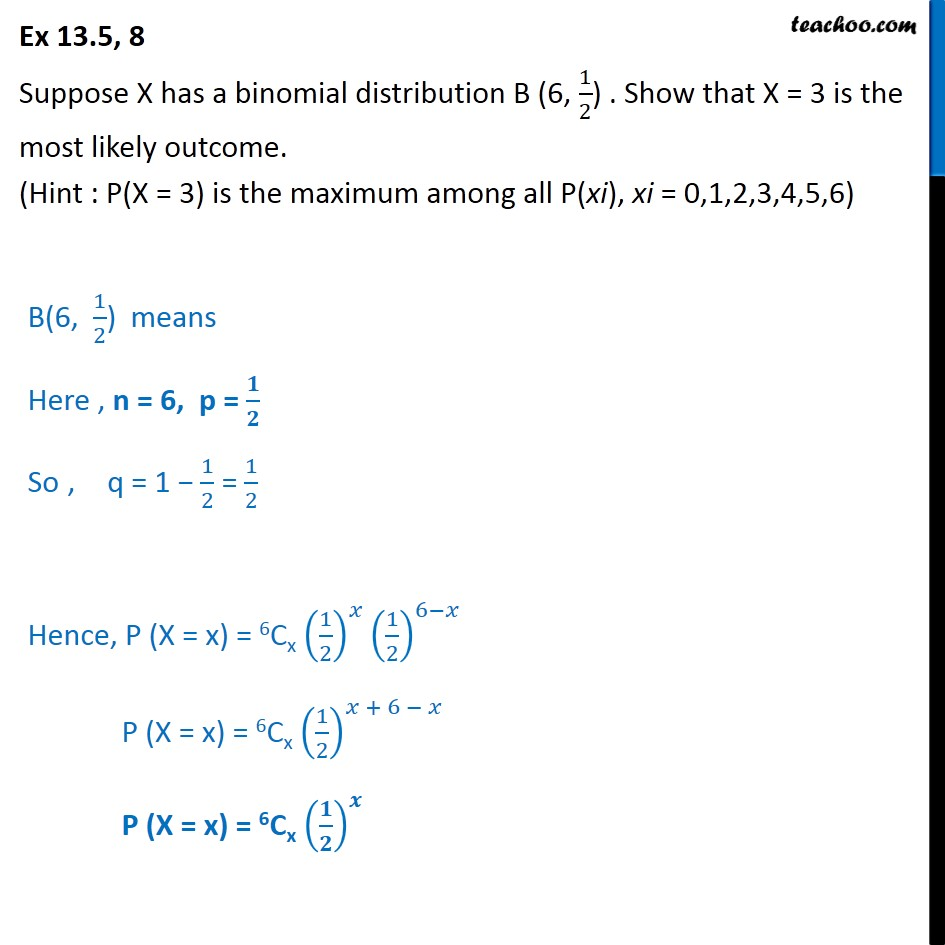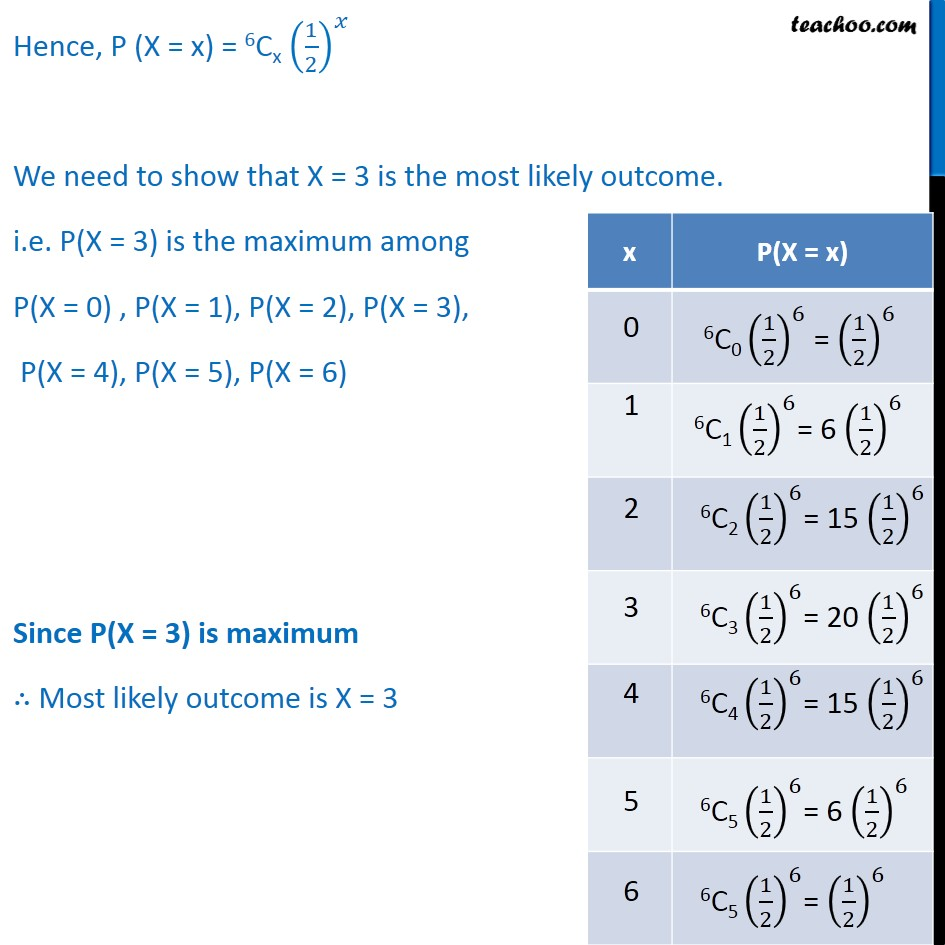Bernoulli Trial

Chapter 13 Class 12 Probability
Serial order wiseLearn in your speed, with individual attention - Teachoo Maths 1-on-1 Class

### Transcript

Question 8 Suppose X has a binomial distribution B (6, 1﷮2﷯) . Show that X = 3 is the most likely outcome. (Hint : P(X = 3) is the maximum among all P(xi), xi = 0,1,2,3,4,5,6) B(6, 1﷮2﷯) means Here , n = 6, p = 𝟏﷮𝟐﷯ So , q = 1 − 1﷮2﷯ = 1﷮2﷯ Hence, P (X = x) = 6Cx 1﷮2﷯﷯﷮𝑥﷯ 1﷮2﷯﷯﷮6−𝑥﷯ P (X = x) = 6Cx 1﷮2﷯﷯﷮𝑥 + 6 − 𝑥﷯ P (X = x) = 6Cx 𝟏﷮𝟐﷯﷯﷮𝒙﷯ Hence, P (X = x) = 6Cx 1﷮2﷯﷯﷮𝑥﷯ We need to show that X = 3 is the most likely outcome. i.e. P(X = 3) is the maximum among P(X = 0) , P(X = 1), P(X = 2), P(X = 3), P(X = 4), P(X = 5), P(X = 6) Since P(X = 3) is maximum ∴ Most likely outcome is X = 3